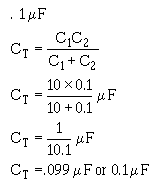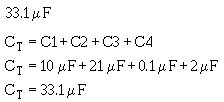Custom SearchANSWERS TO QUESTIONS Q1. THROUGH Q19. A1. A capacitor is a device that stores electrical energy in an electrostatic field. Capacitance is the property of a circuit which opposes changes in voltage. A2. They are polarized from positive to negative. They radiate from a charged particle in straight lines and do not form closed loops. They have the ability to pass through any known material. They have the ability to distort the orbits of electrons circling the nucleus. A3. Toward the positive charge. A4. Two pieces of conducting material separated by an insulator. A5. A farad is the unit of capacitance. A capacitor has a capacitance of 1 farad when a difference of 1 volt will charge it with 1 coulomb of electrons. A6. One microfarad equals 10-6 farad. One picofarad equals 10-12 farad. A7. The area of the plates. The distance between the plates. The dielectric constant of the material between the plates. A8.A9. Hysteresis. Dielectric leakage A10. It is the maximum voltage the capacitor can work without risk of damage. 900 volts. A11. When the capacitor is charging, electrons accumulate on the negative plate and leave the positive plate until the charge on the capacitor is equal to the battery voltage. When the capacitor is discharging, electrons flow from the negatively charged plate to the positively charged plate until the charge on each plate is neutral. A12. At the instant of the initiation of the action. A13. Zero. A14.A15.A16.A17.A18. Electrolytic capacitor. Trimmer capacitor A19. 26 mF or 260,000 pF. 630 pF. 9600 pF.Integrated Publishing, Inc. - A (SDVOSB) Service Disabled Veteran Owned Small Business